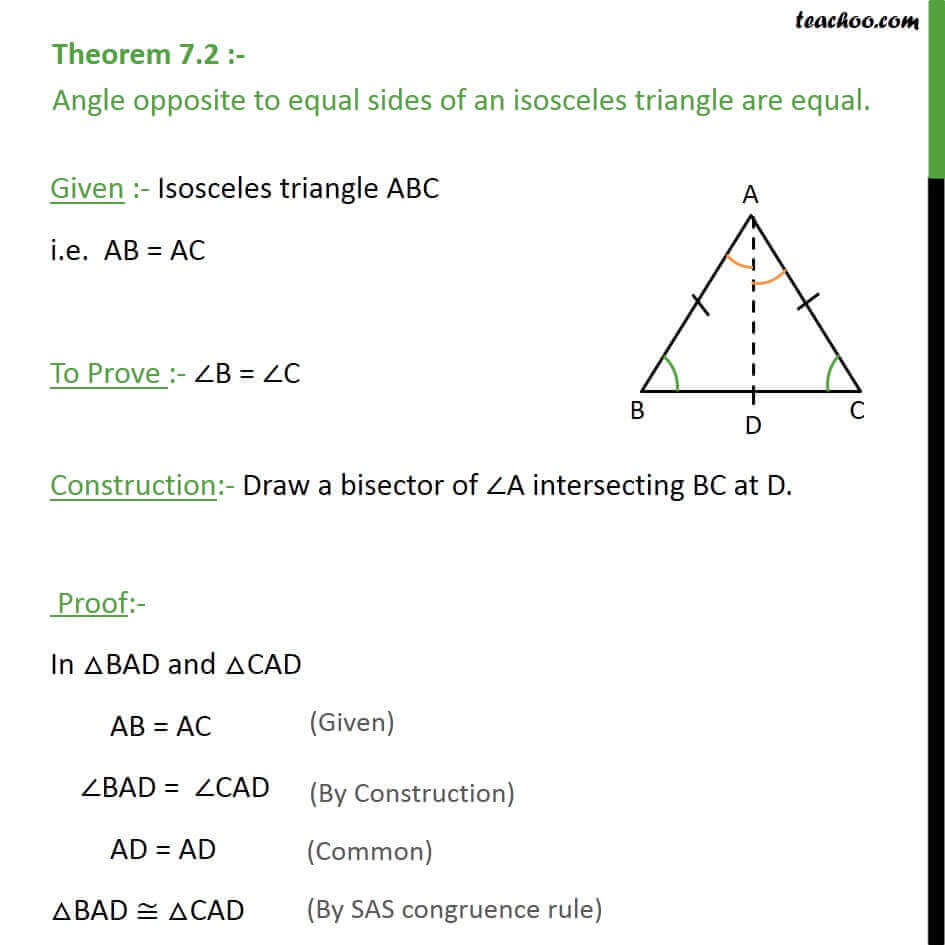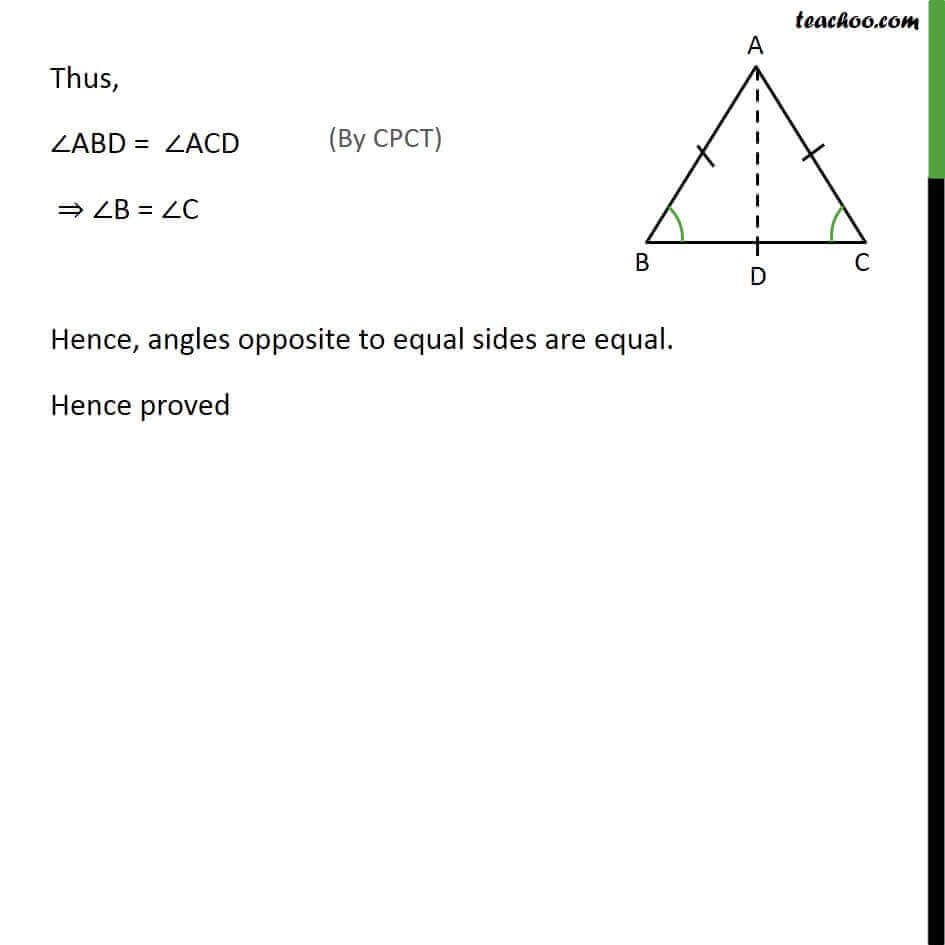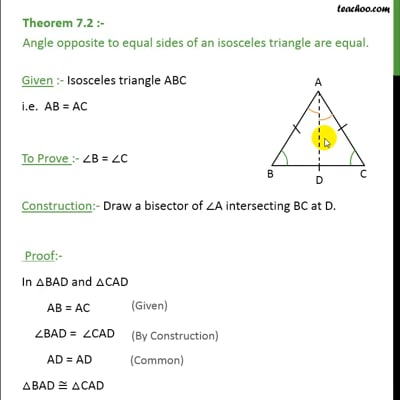Theorems

Chapter 7 Class 9 Triangles
Serial order wiseThis video is only available for Teachoo black users

Solve all your doubts with Teachoo Black (new monthly pack available now!)

### Transcript

Theorem 7.2 :- Angle opposite to equal sides of an isosceles triangle are equal. Given :- Isosceles triangle ABC i.e. AB = AC To Prove :- ∠B = ∠C Construction:- Draw a bisector of ∠A intersecting BC at D. Proof:- In △BAD and △CAD AB = AC ∠BAD = ∠CAD AD = AD △BAD ≅ △CAD Thus, ∠ABD = ∠ACD ⇒ ∠B = ∠C Hence, angles opposite to equal sides are equal. Hence proved